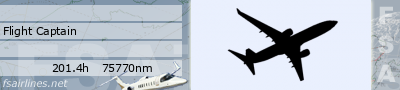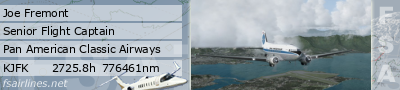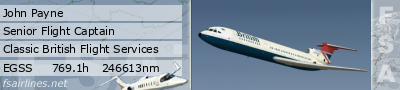## Wrong aircraft type

Please report Bugs and Problems here
Brian Peace
Chief Pilot
Posts: 685
Joined: Tue Jan 22, 2008 3:24 pm
Location: Cardston, Alberta
Contact:

### Re: Wrong aircraft type

Ok here's a new one. Finally got everything ready and loaded newer FSUIPC and everything except aircraft type is ok. Client still reading aircraft type wrong.

I am using FS2002

Aircraft type DC10-30F

Here's it's .CFG file

[fltsim.0]
title=BC NORTH DC-10-30ER

sim=DC1030ER_210
model=
panel=
sound=
texture=
checklists=
description=Aircraft design and textures: FFG group & Kamal S. Kerba\nFlight dynamics: Hervé Sors\nCredits: Ron Freimuth, Prevair Group\n(C) Freeware Flight Group\nVersion 2.10\nREPAINT BRIAN PEACE
ui_manufacturer=BC NORTH
ui_type=DC10 30F
ui_variation=
atc_heavy=1
atc_id=
atc_airline=
atc_flight_number=

[forcefeedback]
gear_bump_nose_magnitude=3000
gear_bump_nose_direction=18000
gear_bump_nose_duration=250000
gear_bump_left_magnitude=2700
gear_bump_left_direction=35500
gear_bump_left_duration=250000
gear_bump_right_magnitude=2700
gear_bump_right_direction=00500
gear_bump_right_duration=250000
ground_bumps_magnitude1=1300
ground_bumps_angle1=8900
ground_bumps_intercept1=3.0
ground_bumps_slope1=0.20
ground_bumps_magnitude2=200
ground_bumps_angle2=9100
ground_bumps_intercept2=1.075
ground_bumps_slope2=0.035
crash_magnitude1=10000
crash_direction1=01000
crash_magnitude2=10000
crash_direction2=9000
crash_period2=75000
crash_duration2=2500000

[General]
performance=Engines: 3 x CF6-50C2 @ 51,800 lb each\nMTOW: 580,000 lb \s MLW: 421,000 lb\nFuel capacity: 41,413 USG (277,434 lb @ 6.7 lb\/USG)\nMax speed: 350 kts IAS or Mach 0.88\nFlaps settings: 5-15-20-25-30-35-50°\nMax operating altitude: 42,000 Ft\nTypical cruise setting: FL310-370 @ Mach 0.82\nRange with typical payload: 7,500 NM \n
atc_type=GEMINI
atc_model=DC10 30F

[WEIGHT_AND_BALANCE]
reference_datum_position= 0.000, 0.000, 0.000
empty_weight_CG_position= 0.000, 0.000, 0.000
max_number_of_stations=50
empty_weight= 302566.000
empty_weight_roll_MOI= 16400000.000
empty_weight_pitch_MOI= 29900000.000
empty_weight_yaw_MOI= 45100000.000
empty_weight_coupled_MOI= 451000.000
max_gross_weight= 580033.092
CG_forward_limit= 0.000
CG_aft_limit= 1.000

[fuel]
Center1= 1.000, 0.000, 0.000, 14528.000, 3.000
Center2= 2.000, 0.000, 0.000, 14735.000, 3.000
LeftMain= -6.100, -27.600, 0.000, 6075.000, 3.000
RightMain= -6.100, 27.600, 0.000, 6075.000, 3.000
fuel_type=2.000000
number_of_tank_selectors=1

[airplane_geometry]
wing_area= 4080.000
wing_span= 165.333
wing_root_chord= 24.667
wing_dihedral= 4.197
wing_incidence= 0.698
wing_twist= -1.494
oswald_efficiency_factor= 0.798
wing_winglets_flag= 0
wing_sweep= 0.000
wing_pos_apex_lon= 6.167
wing_pos_apex_vert= 0.000
htail_area= 1375.000
htail_span= 71.167
htail_pos_lon= -66.250
htail_pos_vert= 0.000
htail_incidence= 0.000
htail_sweep= 0.000
vtail_area= 825.000
vtail_span= 32.749
vtail_sweep= 0.000
vtail_pos_lon= -57.917
vtail_pos_vert= 5.833
elevator_area= 45.000
aileron_area= 20.000
rudder_area= 50.000
elevator_up_limit= 26.001
elevator_down_limit= 26.001
aileron_up_limit= 26.001
aileron_down_limit= 26.001
rudder_limit= 26.001
elevator_trim_limit= 17.189
spoiler_limit= 59.989
spoilerons_available= 0
aileron_to_spoileron_gain= 0.000
min_ailerons_for_spoilerons= 0.000
min_flaps_for_spoilerons= 0.000

[contact_points]
point.0= 1.000, 63.583, 0.000, -11.833, 1181.102, 0.000, 4.521, 75.780, 0.885, 2.500, 0.793, 10.000, 10.000, 0.000, 0.000, 0.000
point.1= 1.000, -8.750, -17.500, -11.833, 1574.803, 1.000, 4.521, 0.000, 0.899, 2.500, 0.469, 14.900, 14.900, 2.000, 0.000, 0.000
point.2= 1.000, -8.750, 17.500, -11.833, 1574.803, 2.000, 4.521, 0.000, 0.899, 2.500, 0.469, 15.100, 15.100, 3.000, 0.000, 0.000
point.3= 2.000, -43.667, -82.667, 4.417, 787.402, 0.000, 0.000, 0.000, 0.000, 0.000, 0.000, 0.000, 0.000, 5.000, 0.000, 0.000
point.4= 2.000, -43.667, 82.667, 4.417, 787.402, 0.000, 0.000, 0.000, 0.000, 0.000, 0.000, 0.000, 0.000, 6.000, 0.000, 0.000
point.5= 2.000, -77.833, 0.000, 6.083, 787.402, 0.000, 0.000, 0.000, 0.000, 0.000, 0.000, 0.000, 0.000, 9.000, 0.000, 0.000
point.6= 2.000, 64.917, 0.000, 6.083, 787.402, 0.000, 0.000, 0.000, 0.000, 0.000, 0.000, 0.000, 0.000, 4.000, 0.000, 0.000
static_pitch= 0.000
static_cg_height= 11.414

[GeneralEngineData]
engine_type= 1
Engine.0= 10.667, -26.833, -5.500
Engine.1= -69.500, 0.000, 17.333
Engine.2= 10.667, 26.833, -5.500
fuel_flow_scalar= 1.000

[TurbineEngineData]
fuel_flow_gain= 0.002
inlet_area= 87.000
rated_N2_rpm= 29920.000
static_thrust= 51800.000
afterburner_available= 0
reverser_available= 1

[Reference Speeds]
flaps_up_stall_speed= 170.000
full_flaps_stall_speed= 136.000
cruise_speed= 497.985

[keyboard_response]
elevator=100.000000,200.000000
aileron=100.000000,200.000000
rudder=100.000000,200.000000

[Views]
eyepoint=86.250205,-1.486631,7.830442

[autopilot]
autopilot_available=1
flight_director_available=1
default_vertical_speed=1800.000000
autothrottle_available=1
autothrottle_arming_required=1
autothrottle_takeoff_ga=1
autothrottle_max_rpm=90.000000
pitch_takeoff_ga=8.000000

[direction_indicators]
direction_indicator.0=3,0

[Flaps.0]
type= 1
span-outboard= 0.500
extending-time= 20.000
system_type= 1
damaging-speed= 0.000
blowout-speed= 0.000
lift_scalar= 1.000
drag_scalar= 1.000
pitch_scalar= 1.000
flaps-position.0= 0.000, 0.000
flaps-position.1= 7.143, 0.000
flaps-position.2= 14.286, 0.000
flaps-position.3= 21.429, 0.000
flaps-position.4= 28.571, 0.000
flaps-position.5= 35.714, 0.000
flaps-position.6= 42.857, 0.000
flaps-position.7= 50.000, 0.000
MAINLAND PILOTS CLUB
http://bcnorth.tripod.com/joefremont
FSAirlines Developer
Posts: 2296
Joined: Tue May 16, 2006 5:46 am
Location: KSFO

### Re: Wrong aircraft type

The weight check does not seam to work for FS2002 and that will be fixed in the next release.Brian Peace
Chief Pilot
Posts: 685
Joined: Tue Jan 22, 2008 3:24 pm
Location: Cardston, Alberta
Contact:

### Re: Wrong aircraft type

In the news today.... BC North pilot Brian Peace was grounded. Some say he had the smell of alchohol on his breath, but others say it was becuase of some "client" he was murmuring about.When can I expect the new release? Im dyin to try out FSAMAINLAND PILOTS CLUB
http://bcnorth.tripod.com/davebrindley

### Re: Wrong aircraft type

Please can someone help... I am getting "Wrong aircraft type" error in the client (ver 1.0.2) I have 2 Pilatus PC-6 aircraft at FAKN and we have the FSD payware Pilatus PC-6 Landplane running in FS2004. Aircraft.cfg weights and balances are as follows:

[WEIGHT_AND_BALANCE]
empty_weight = 2800.0 // (pounds)
max_gross_weight=4850.5

max_number_of_stations = 20

Quantum
Posts: 1439
Joined: Sat Apr 29, 2006 8:23 pm
Location: UK

### Re: Wrong aircraft type

Hi,

I just checked the PC-6 data and the FSA database was slightly out, but your weights are way out. Try editing your max gross weight to ' max_gross_weight=6106 ' and I reckon you will be good to go. The pax load has been reduced to 10 as the previous number of 11 was including the pilot.

Regards

John
CEO - Classic British Flight Services
Classic aircraft on Classic routesBrian Peace
Chief Pilot
Posts: 685
Joined: Tue Jan 22, 2008 3:24 pm
Location: Cardston, Alberta
Contact:

### Re: Wrong aircraft type

So far so good with v1.03 and FS2002. We're in the airMAINLAND PILOTS CLUB
http://bcnorth.tripod.com/davebrindley

### Re: Wrong aircraft type

Quantum wrote:Hi,

I just checked the PC-6 data and the FSA database was slightly out, but your weights are way out. Try editing your max gross weight to ' max_gross_weight=6106 ' and I reckon you will be good to go. The pax load has been reduced to 10 as the previous number of 11 was including the pilot.
Thanks Quantum... Will edit it and give it a try. Will let you know.

Ticket Agent
Posts: 7
Joined: Wed Apr 23, 2008 3:41 am
Contact:

### Re: Wrong aircraft type

Hey guys,

well heres whats going on. I filed a flight with the DH8D and when I went to fly it in FS2004, the wrong aircraft popped up. er it's long but heres my full aircraft .cfg;

[fltsim.0]
title= Dash 8-Q400
sim=Dash8Q400
model=
panel=
sound=
texture=2
checklists=
ui_manufacturer=Executive Virtual Airlines
ui_type=
description=Dreamwings Dash8 Q400

For Executive Virtual Airlines
atc_id=C-GUYT
atc_heavy=0
atc_flight_number=8294

[General]
performance=Model Designer: Tommy Hansson\nFDE: John Cillis\n\nFlightcrew:\t2 Pilots and 2-3 Cabin Crew\nPax:\t70\nMax cruising speed:\t360kts\nMax certificated ceiling:\t25,000ft\nMax range with 70 passengers and reserves:\t1362nm\n\nWing span\t28.42m (93ft 3in)\nLength\t32.84m (107ft 9in)\nHeight\t8.38m (27ft 5in)\nWing area\t63.1m2 (679.0sq ft).
atc_type=Bombardier
atc_model=DHC84
editable=1

[WEIGHT_AND_BALANCE]
max_gross_weight=64,500 // (pounds)
empty_weight=37,888 // (pounds)

reference_datum_position=0, 0, 0 // (feet) distance from FlightSim Reference position: (1/4 chord, centerline, waterline)
empty_weight_CG_position=0, 0, 0 // (feet) longitudinal, lateral, vertical distance from specified datum
max_number_of_stations=8

//Cabin

//Baggage Cmpt

;Moments of Inertia
empty_weight_pitch_MOI=311701
empty_weight_roll_MOI=361201
empty_weight_yaw_MOI=361691
empty_weight_coupled_MOI=0

//0 Class <0=none,1=wheel, 2=scrape, 3=float>
//1 Longitudinal Position (feet)
//2 Lateral Position (feet)
//3 Vertical Position (feet)
//4 Impact Damage Threshold (Feet Per Minute)
//5 Brake Map (0=None, 1=Left, 2=Right)
//7 Steer Angle (degrees)
//8 Static Compression (feet) (0 if rigid)
//9 Max/Static Compression Ratio
//10 Damping Ratio (0=Undamped, 1=Critically Damped)
//11 Extension Time (seconds)
//12 Retraction Time (seconds)
//13 Sound Type
//14 Airspeed limit for retraction (KIAS)
//15 Airspeed that gear gets damage at (KIAS)

[contact_points]

point.0=1, 47.7, 0.00, -6.8, 1500.00, 0.000, 1.161, 60.000, 0.075, 2.500, 0.987, 5.000, 5.000, 0.000, 0.000, 0.000
point.1=1, -2.0, -12.99, -7.3, 1574.803, 1.000, 1.161, 0.000, 0.215, 2.500, 0.642, 5.000, 5.000, 2.000, 0.000, 0.000
point.2=1, -2.0, 12.99, -7.3, 1574.803, 2.000, 1.161, 0.000, 0.215, 2.500, 0.642, 5.000, 5.000, 3.000, 0.000, 0.000
point.3=2, 5.0, -47.8, 6.3, 1200, 0, 0.000, 0.0, 0.000, 0.0, 0.0, 0.0, 0.0, 5
point.4=2, 5.0, 47.8, 6.3, 1200, 0, 0.000, 0.0, 0.000, 0.0, 0.0, 0.0, 0.0, 6
point.5=2, -33.6, 0.00, 1.22, 1200, 0, 0.000, 0.0, 0.000, 0.0, 0.0, 0.0, 0.0, 9
point.6=2, 40.0, 0.00, 1.22, 1200, 0, 0.000, 0.0, 0.000, 0.0, 0.0, 0.0, 0.0, 4

max_number_of_points=21

static_pitch=-0.4 //degrees, pitch when at rest on the ground (+=Up, -=Dn)
static_cg_height=7.1 //feet, altitude of CG when at rest on the ground
gear_system_type=0

[Views]
eyepoint=43.400, 0, 1.7 //(feet) longitudinal, lateral, vertical distance from reference datum

[GeneralEngineData]
engine_type=5 //0=Piston, 1=Jet, 2=None, 3=Helo-Turbine, 4=Rocket, 5=Turboprop
Engine.0=0, -13.3, 2.7 //(feet) longitudinal, lateral, vertical distance from reference datum
Engine.1=0, 13.3, 2.7 //(feet) longitudinal, lateral, vertical distance from reference datum
fuel_flow_scalar=1.5
min_throttle_limit=-0.250000

[TurbineEngineData]
fuel_flow_gain=0.011000
inlet_area=.25
rated_N2_rpm=29920.000000
static_thrust=200.000000
afterburner_available=0

[propeller]
thrust_scalar=1.0 //Propeller thrust scalar
propeller_type=0 //0=Constant Speed, 1=Fixed Pitch
propeller_diameter=13.5 //Propeller Diameter, (feet)
propeller_moi=77 //Propeller moment of inertia
beta_max=45 //Maximum blade pitch angle for constant speed prop, (degrees)
beta_min=12 //Minimum blade pitch angle for constant speed prop, (degrees)
min_gov_rpm=25520 //Miminum governed RPM
prop_tc=0.0025 //Prop time-constant
gear_reduction_ratio=17.0 //Propeller gear reduction ratio
fixed_pitch_beta=0 //Fixed pitch angle of fixed pitch prop, (degrees)
low_speed_theory_limit=80 //Speed at which low speed theory becomes blended in (feet/second)
prop_sync_available=1 //Prop synchronization available? 0=FALSE, 1=TRUE
prop_deice_available=1 //Prop de-icing available? 0=FALSE, 1=TRUE
prop_feathering_available=1 //Prop feathering available? 0=FALSE, 1=TRUE
prop_auto_feathering_available=1 //Prop auto-feathering available? 0=FALSE, 1=TRUE
min_rpm_for_feather=700 //Minimum RPM for prop feathering
beta_feather=79.3 //Feathering pitch angle (degrees)
power_absorbed_cf=0.9 //Coefficient of friction for power absorbed by propeller
defeathering_accumulators_available=0 //Defeathering accumulators available? 0=FALSE, 1=TRUE
prop_reverse_available=1 //Prop reverse available? 0=FALSE, 1=TRUE
minimum_on_ground_beta=1.0 //Miminum pitch angle on ground, (degrees)
minimum_reverse_beta=-14.0 //Minimum pitch angle in reverse, (degrees)
rotation=1,1

[turboprop_engine]
power_scalar=1.66 //Scalar on Turboprop power
maximum_torque=4500 //Maximum torque available (ft-lbs)

[fuel]
LeftMain=0, -9.1, 3.9, 886.0, 0.0 //Longitudinal (feet), Lateral (feet), Vertical (feet), Usable(gallons), Unusable (gallons)
RightMain=0, 9.1, 3.9, 886.0, 0.0 //Longitudinal (feet), Lateral (feet), Vertical (feet), Usable(gallons), Unusable (gallons)
fuel_type=2 //Fuel type: 1 = Avgas, 2 = JetA
number_of_tank_selectors=1
electric_pump=0

[flight_tuning]
cruise_lift_scalar=1.0
parasite_drag_scalar=.88
induced_drag_scalar=.88
elevator_effectiveness=1.0
aileron_effectiveness=1.0
rudder_effectiveness=1.0
pitch_stability=3.0
roll_stability=2.0
yaw_stability=2.0
elevator_trim_effectiveness=3.0
aileron_trim_effectiveness=1.0
rudder_trim_effectiveness=1.0

[electrical]
flap_motor=0, 5 , 17.0
gear_motor=0, 5 , 17.0
autopilot=0, 5 , 17.0
avionics_bus=0, 10, 17.0
avionics=1, 5 , 17.0
pitot_heat=0, 2 , 17.0
marker_beacon=1, 2 , 17.0
gear_warning=0, 2 , 17.0
fuel_pump=0, 5 , 17.0
starter1=0, 20, 17.0
light_nav=0, 5 , 17.0
light_beacon=0, 5 , 17.0
light_landing=0, 5 , 17.0
light_taxi=0, 5 , 17.0
light_strobe=0, 5 , 17.0
light_panel=0, 5 , 17.0

[flaps.0]
type=1 // 1 - tail, 2 - lead
span-outboard=0.8 // 0.0 .. 1.0
extending-time=5 // seconds
flaps-position.0=0 // degrees, KIAS
flaps-position.1=10 // degrees, KIAS
flaps-position.2=30 // degrees, KIAS
flaps-position.3=40 // degrees, KIAS
damaging-speed=250 // KIAS
blowout-speed=300 // KIAS

[EFFECTS]
wake=fx_wake
water=fx_spray
dirt=fx_tchdrt
concrete=fx_sparks
touchdown=fx_tchdwn, 1

[airplane_geometry]
wing_area=679.0 //Square feet
wing_span=93.25 //Feet
wing_root_chord=7.25 //Feet
wing_dihedral=2.5 //Degrees
wing_incidence=-2.5 //Degrees
wing_twist=2.5 //Degrees
oswald_efficiency_factor=.7 //Measure of lift effeciency of wing
wing_winglets_flag=0 //Are winglets available?
wing_pos_apex_lon=-25.0 //Feet, longitudinal distance from reference point, negative going aft
wing_pos_apex_vert=0.0 //Feet, vertical distance from reference point, positive going up
htail_area=180.0 //Square feet
htail_span=26.0 //Feet
htail_pos_lon=-58.9 //Feet, longitudinal distance from reference point, negative going aft
htail_pos_vert=0.0 //Feet, vertical distance from reference point, positive going up
htail_incidence=1.0 //Degrees
htail_sweep=8.0 //Degrees, horizontal tail leading edge
vtail_area=152.0 //Square feet
vtail_span=11.7 //Feet, tip to body
vtail_sweep=30.0 //Degrees, vertical tail leading edge
vtail_pos_lon=-54.9 //Feet, longitudinal distance from reference point, negative going aft
vtail_pos_vert=3.4 //Feet, vertical distance from reference point, positive going up
elevator_area=53.5 //Square feet
aileron_area=12.1 //Square feet
rudder_area=46.4 //Square feet
elevator_up_limit=17.0 //Degrees
elevator_down_limit=15.5 //Degrees
aileron_up_limit=18.0 //Degrees
aileron_down_limit=18.0 //Degrees
rudder_limit=30.0 //Degrees
elevator_trim_limit=15.0 //Degrees
spoiler_limit=60.0 //Degrees
spoiler_limit= 60.000
spoilerons_available=1 //Spoilerons Available?
aileron_to_spoileron_gain=3.000 //Aileron to spoileron gain
min_ailerons_for_spoilerons=9.969 //Degrees
min_flaps_for_spoilerons=0 //Minimum flap handle position when spoilerons activate

[Reference Speeds]
flaps_up_stall_speed=92.0 //Knots True (KTAS)
full_flaps_stall_speed=72.0 //Knots True (KTAS)
cruise_speed=350.0 //Knots True (KTAS)
max_indicated_speed=265.000000
max_mach=0.60

[keyboard_response]
//Breakpoint speeds (knots) on the keyboard increments of control surfaces.
//Speed at which the incremenet is reduced to 1/2 and 1/8 respectively.
elevator=150.0, 250.0
aileron=150.0, 250.0
rudder=150.0, 250.0

[autopilot]
use_no_default_pitch=1
use_no_default_bank=1
autopilot_available=1
flight_director_available=1
default_vertical_speed=1000.0
autothrottle_available=1
pitch_takeoff_ga=8.000000
default_pitch_mode=0
default_bank_mode=0
max_pitch=10.000000
max_pitch_acceleration=1.000000
max_pitch_velocity_lo_alt=2.000000
max_pitch_velocity_hi_alt=1.500000
max_pitch_velocity_lo_alt_breakpoint=20000.000000
max_pitch_velocity_hi_alt_breakpoint=28000.000000
max_bank=25.000000
max_bank_acceleration=1.800000
max_bank_velocity=3.000000
max_throttle_rate=0.100000
nav_proportional_control=9.000000
nav_integrator_control=0.250000
nav_derivative_control=0.000000
nav_integrator_boundary=2.500000
nav_derivative_boundary=0.000000
gs_proportional_control=9.520000
gs_integrator_control=0.260000
gs_derivative_control=0.000000
gs_integrator_boundary=0.700000
gs_derivative_boundary=0.000000
yaw_damper_gain=1.000000
autothrottle_arming_required=0
autothrottle_takeoff_ga=0
autothrottle_max_rpm=90.000000

[forcefeedback]
stick_shaker_magnitude=5000 ; 0 - 10000
stick_shaker_direction=0 ; 0 - 35999 degrees
stick_shaker_period=111111 ; in microseconds
gear_bump_nose_magnitude=3000 ; 0 - 10000
gear_bump_nose_direction=18000 ; 0 - 35999 degrees
gear_bump_nose_duration=250000 ; in microseconds
gear_bump_left_magnitude=2700 ; 0 - 10000
gear_bump_left_direction=9000 ; 0 - 35999 degrees
gear_bump_left_duration=250000 ; in microseconds
gear_bump_right_magnitude=2700 ; 0 - 10000
gear_bump_right_direction=27000 ; 0 - 35999 degrees
gear_bump_right_duration=250000 ; in microseconds
ground_bumps_magnitude1=1300 ; 0 - 10000
ground_bumps_angle1=08900 ; 0 - 35999 degrees
ground_bumps_intercept1=3.0
ground_bumps_slope1=0.20
ground_bumps_magnitude2=200 ; 0 - 10000
ground_bumps_angle2=09100 ; 0 - 35999 degrees
ground_bumps_intercept2=1.075
ground_bumps_slope2=0.035
crash_magnitude1=10000 ; 0 - 10000
crash_direction1=01000 ; 0 - 35999 degrees
crash_magnitude2=10000 ; 0 - 10000
crash_direction2=9000 ; 0 - 35999 degrees
crash_period2=75000 ; in microseconds
crash_duration2=2500000 ; in microseconds

[LIGHTS]
light.0=3, 5.0, -47.8, 6.3, fx_navred ,
light.1=3, 5.0, 47.8, 6.3, fx_navgre ,
light.2=2, 5.3, 47.8, 6.3, fx_strobe ,
light.3=2, 5.3, -47.8, 6.3, fx_strobe ,
light.4 = 3, -50.069, -0.016, 19.965, fx_strobeh ,
light.5 = 1, 17.875, -0.007, 4.644, fx_beacon ,
light.6 = 2, -59.003, 0, 18.829, fx_navwhih ,
light.7=4, -7.91, 0.00, 2.64, fx_vclight,

[direction_indicators]
direction_indicator.0=3,0

[brakes]
toe_brakes_scale=1.062532
parking_brake=1

[gear_warning_system]
gear_warning_available=1
pct_throttle_limit=0.099976
flap_limit_power=30.022988
flap_limit_idle=15.011494

[hydraulic_system]
electric_pumps=0
engine_map=1,1,0,0
normal_pressure=3000.000000

[stall_warning]
type=1

[attitude_indicators]
attitude_indicator.0=1

[turn_indicators]
turn_indicator.0=1,0

[exits]
number_of_exits=3
exit_rate.0=0.25 ;Percent per second
exit_rate.1=0.35 ;Percent per second
exit_rate.2=0.30 ;Percent per second

[TailHook]
tailhook_length=1 ;(feet)
tailhook_position = 0.0, 0.0, 0.0 ;(feet) longitudinal, lateral, vertical positions from datum

[folding_wings]
wing_fold_system_type = 4 ;0=None
fold_rates = 0.05,0.05 ;Percent per second

// Radio Type=availiable, standby frequency, has glide slope
Audio.1=1
Com.1=1, 1
Com.2=1, 1
Nav.1=1, 1, 1
Nav.2=1, 1, 1
Transponder.1=1
Marker.1=1http://fly-virtualea.weebly.comjoefremont
FSAirlines Developer
Posts: 2296
Joined: Tue May 16, 2006 5:46 am
Location: KSFO

### Re: Wrong aircraft type

Its a total shot in the dark because the weights look good, but maybe you should try removing the comma's in the numbers for max_gross_weight and empty_weight.Ticket Agent
Posts: 7
Joined: Wed Apr 23, 2008 3:41 am
Contact:

### Re: Wrong aircraft type

I tried still a no gohttp://fly-virtualea.weebly.comCAPFlyer
Chief Pilot
Posts: 3045
Joined: Mon Nov 07, 2005 2:49 am
Location: Lancaster, Texas, USA
Contact:

### Re: Wrong aircraft type

The Commas were definitely an issue, but the "ATC_MODEL" is also a problem as it's invalid (it's shown as DHC84, which is invalid and too many even for FS2004). Try changing it to DH8D and see if that fixes the problem.Ticket Agent
Posts: 7
Joined: Wed Apr 23, 2008 3:41 am
Contact:

### Re: Wrong aircraft type

You Guys are awesome!you also saved my VA

Thank you.http://fly-virtualea.weebly.comStan
Chief Pilot
Posts: 650
Joined: Thu Nov 09, 2006 11:57 pm
Location: Manchester.UK

### Re: Wrong aircraft type

Re ilyushin 96 alter cfg.from this:-

[General]
atc_type=Ilyushin
atc_model=IL-96
editable=1
performance=Length:59 m\nWing span: 48 m\nWeight: 208 000 kg (MTOW)\nPower: Kuznetsov NK-86 x4\nPassengers: 350\nRange: 5000 km (2600 nm)

to

[General]
atc_type=Ilyushin
atc_model=IL96
editable=1
performance=Length:59 m\nWing span: 48 m\nWeight: 208 000 kg (MTOW)\nPower: Kuznetsov NK-86 x4\nPassengers: 350\nRange: 5000 km (2600 nm)Yours StanTicket Agent
Posts: 7
Joined: Wed Apr 23, 2008 3:41 am
Contact:

### Re: Wrong aircraft type

o boy

well Idon't know if I should start a new thread or not but I'm now having problems with weight and balence in FS. My aircraft is way too light or way too heavy on takeoff. I 've already posted the cfg file ( read previous posts) .

can anyone help?

tyhttp://fly-virtualea.weebly.comQuantum
Posts: 1439
Joined: Sat Apr 29, 2006 8:23 pm
Location: UK

### Re: Wrong aircraft type

well Idon't know if I should start a new thread or not but I'm now having problems with weight and balence in FS. My aircraft is way too light or way too heavy on takeoff. I 've already posted the cfg file ( read previous posts) .

can anyone help?

ty
Hi,

From your previous post, the aircraft is now recognised by the client. Apart from refuelling your aircraft and checking the aircraft type, that is all the client will do. Once you are in the aircraft, the client will then restrict your revenue load according to what fuel you take onboard. When I say restricted, this means that this is the amount you will get paid for. The client WILL alter your fuel load in the sim via FSUIPC but it will NOT alter the payload in sim. It is up to you to manually adjust your payload as required.

Regards

John
CEO - Classic British Flight Services
Classic aircraft on Classic routes